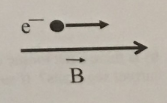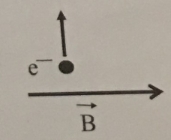# Problem: a). In the figure below, an electron is moving parallel to a uniform magnetic field in the direction shown. Is there a force on the electron; if so what direction does it point? Explain your reasoning. b). In the figure below, an electron is moving perpendicular to a uniform magnetic field in the direction shown. Is there a force on the electron; if so what direction does it point? Explain your reasoning.

###### FREE Expert Solution

The force on the electron is:

$\overline{){\mathbf{F}}{\mathbf{=}}{\mathbf{q}}{\mathbf{\left(}}{\mathbf{v}}{\mathbf{×}}{\mathbf{B}}{\mathbf{\right)}}{\mathbf{=}}{\mathbf{q}}{\mathbf{v}}{\mathbf{B}}{\mathbf{s}}{\mathbf{i}}{\mathbf{n}}{\mathbf{\theta }}}$

θ = 0° since the electron is moving parallel to the uniform magnetic field.

100% (180 ratings)###### Problem Details

a). In the figure below, an electron is moving parallel to a uniform magnetic field in the direction shown. Is there a force on the electron; if so what direction does it point? Explain your reasoning.b). In the figure below, an electron is moving perpendicular to a uniform magnetic field in the direction shown. Is there a force on the electron; if so what direction does it point? Explain your reasoning.